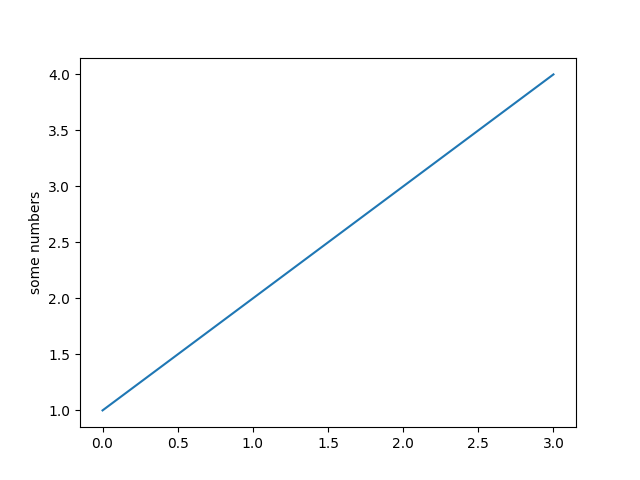# Pyplot Simple¶

A most simple plot, where a list of numbers is plotted against their index.

import matplotlib.pyplot as plt
plt.plot([1, 2, 3, 4])
plt.ylabel('some numbers')
plt.show()